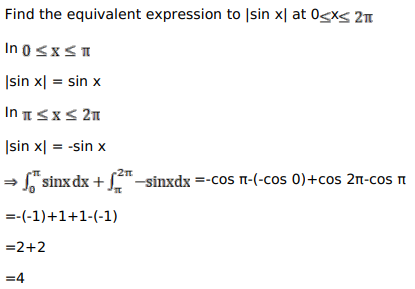# Mark against the correct answer in the following:Question:

Mark $(\sqrt{)}$ against the correct answer in the following:

$\int_{0}^{2 \pi}|\sin x| d x=?$

A. 2

B. 4

C. 1

D. none of these

Solution: